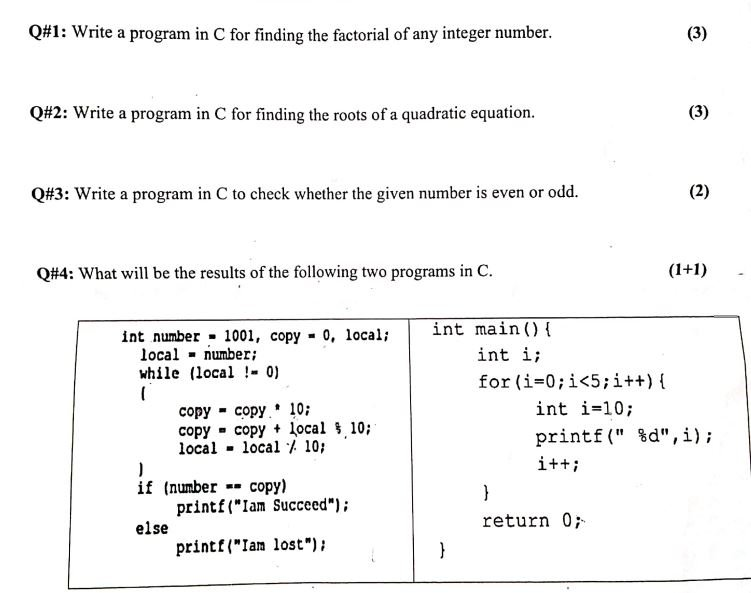# Introduction to computer programming past question paper

– Introduction to computer programming –Computer Science all subject past paper  Introduction Introduction to computer programming-Computer Graphics  Course  computer programming Project idea – ICT  MCQ -Introduction to computer programming Interview Question  –Computer Science all courses -Technology –University Past Paper -Programming language –Question paperold paper

## computer programming Mid term past question paper## Introduction to computer programming Sessional 2 past paper

Q.No.1: Which Statement is true or false?

2. If a 4; and b=9;, then after the statement a = b; the value of b is still 9.

3. If x=5. After the statement y=x++; executes, y is 5 and x is 6. ”

4.Every if statement mush has a corresponding else statement. 5. It is possible that the body of a while loop may not execute at all.

6. If N is even number then (N% 2)=1.

7.

The staternent return is not a reserved word.

8.

The statement print) takes in from keyboard…

9. The number 5 is a floating-point number.

10.

The statement if execute two statements after itself.

Q.No.2: (a) Write a program to find the determinant of matrix of order 3×3,

(4+4)

(b) Write a program to find maximum, minimum in the one-dimensional array by using pointers.

Q.No.3: (a) Find the dot and cross products of any two vectors by using C language.

(4+4)

(b) Write a program to convert given one-dimensional array into reverse order.

Q.1 -How ceil() function works in C? write a function that breaks float to its binary significand

Q.2 Write a program to take 10 integer input from the user suppose array look like this

2

1

6

4

7

9

0

1

2

8

Add two consecutive index number and save into another array with the length of five the other array

will look like this

3

10

16

1

10

No.2: (a) Write a program to find the determinant of matrix of order 3×3. (b) Write a program to find maximum, minimum in the one-dimensional array by using

(4+4)

pointers. No-3: (a) Find the dot and cross products of any two vectors by using C language.

(4+4)

#Introduction to computer programming #Computer Science all subject past paper  Introduction Introduction to computer programming #Computer Graphics  Course  #Introduction to computer programming Project idea  # Introduction to computer programming MCQ  #Introduction to computer programming Interview Question  #Computer Science all courses #Technology #University Past Paper #Programming language  #Question paper  #old paper

Scroll to Top